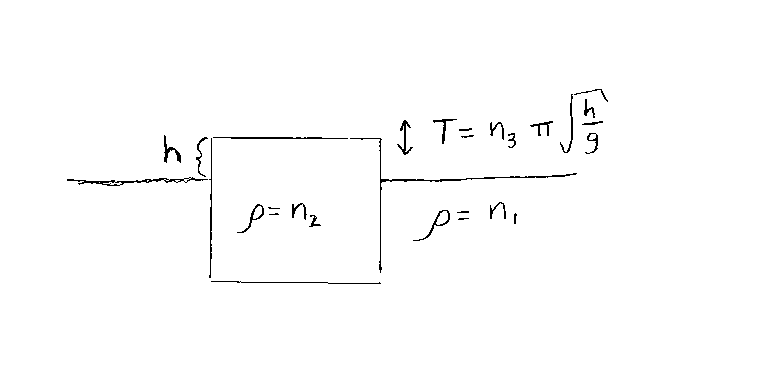Problem C20: A block is floating in a fluid. The density of the fluid is n1. The density of the block is n2. The top of the block is a distance h above the surface of the fluid as shown below. The block is given a slight push downward and oscillates up and down at the fluid's surface. What is the period T of oscillation of the block? If T=n3π √h/g, what is n3? Note that n1 and n2 have the same units of density. (The density of water is 100 in these units.) n3 is unitless. g is the acceleration due to gravity.n1 = n2 = Input n3:

If you are currently in my class, you can record your grade by entering your name and student ID number (without the leading zeros) below and clicking on "record grade".
 First Name = Last Name = ID = Problem: